de-Broglie matter waves: After Einstein's photoelectric theory and Compton's X-ray experiment, light had to be viewed as having both particle and wave properties, but massive particles such as electrons were still widely considered completely distinct from waves. However, puzzling questions about the
particle interpretation of electrons were already developing as early as 1913 with Niels Bohr's model of the Hydrogen atom. Bohr had succeeded in theoretically explaining
the Rydberg formula for the emission specturm of Hydrogen by assuming that the angular momentum of the orbiting electron was restricted to integer multiples of Planck's constant h. Despite its impressive results, Bohr's model had many shortcomings. It was inconsistent with several other observations, and theoretically provided no justi cation for the quantization assumption. This inspired Louis de Broglie to propose the idea that all particles possess an associated matter wave.

...the determination of the stable motions of the electrons in the atom involves whole numbers, and so far the only phenomena in which whole numbers were involved in physics were those of interference and of eigenvibrations. That suggested the idea to me that electrons

He may have also been encouraged to take this leap by Einstein's work on the photoelectric effect.

The most famous equation to emerge from de Broglie's work was his relation between the momentum of a particle and the wavelength of its matter wave. Such a relation was already known for light quanta. By combining the Planck-Einstein equation, E = hv, with the phase velocity formula,  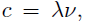, and the result from electromagnetism. E = pc (based on Poynting's Theorem), one can derive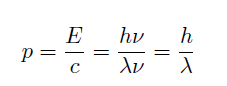In 1923, de Broglie suggested that if matter waves existed, they would also obey this equation.  He started by making the assumption, now believed to be false, that light quanta have some very small but non-zero mass. Under this assumption, he equated the energy of a light quantum given by the Planck-Einstein equation, E = hv, with the relativistic energy of a massive particle given by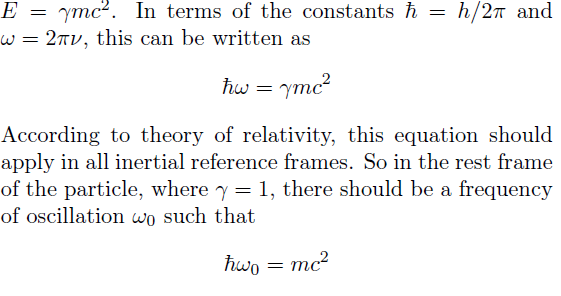In this frame the particle is not moving, so this oscillation would presumably look like a localized standing wave. Now suppose we boost to a reference frame that is moving at velocity v1 with respect to the particle. The particle remains untouched, so its matter wave cannot have changed. However, due to relativistic time-dilation, the apparent frequency of oscillation of the standing wave will be reduced to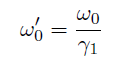If we were to measure the frequency of the wave from this frame by counting the number of crests that pass by per second, we would not get !00 . The reason is that the wave crests will be passing by at a higher rate due to our motion relative to the standing wave. Essentially we are traveling through the wave form as it is oscillating in place. The actual frequency of the wave in our new reference frame is still given by the Planck-Einstein equation. Thus, the relation between the frequency of the wave ω1, and the apparent frequency of its internal oscillationω' 0 , is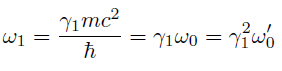Now consider the value of the wave at the center of the light quantum, Ψ(t), still observing from our moving frame. As implied by the de nition of ω' 0 , the value will  ,oscillate sinusoidally according to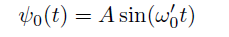provided a suitable choice for the zero point of time. Even though the wave has to be con ned to a nite region, the closer we zoom in on the center, the more
it will look like an in nite monochromatic plane wave, since we are assuming a light quantum with a de nite frequency. The frequency of this monochromatic wave is what we measure by counting passing crests, namely ω1. Therefore, we can approximate the value of the wave near the center of the quantum as